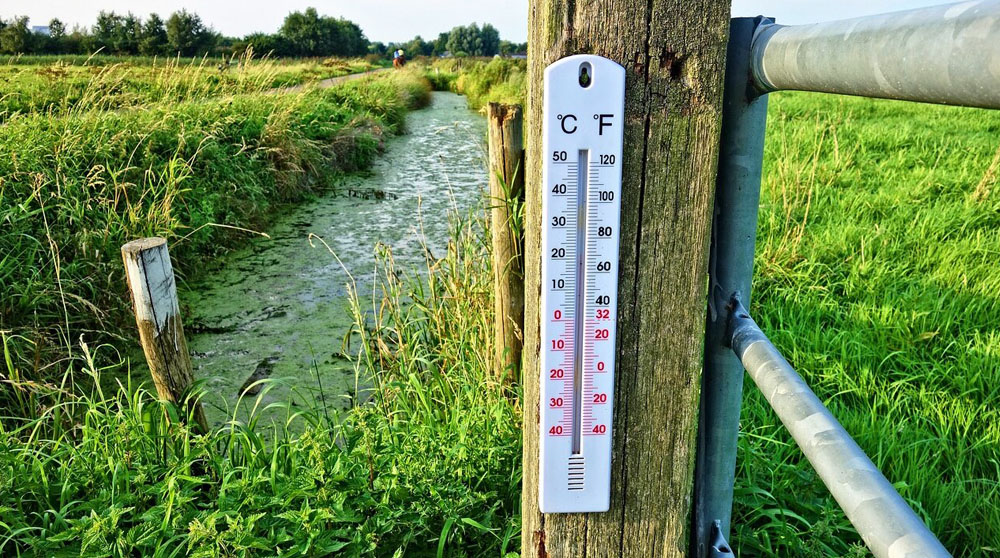# Temperature scale - Celsius, Fahrenheit, Kelvin

Sook ShinIn everyday life and scientific work, people use several temperature scales, the most well-known of which are degrees Celsius, which is the international format in the International System of Units, and degrees Fahrenheit, which is used in everyday life by people living in the United States.

## Kelvin scale

Thermodynamics uses the Kelvin scale, in which temperature is counted from absolute zero (the state corresponding to the minimum theoretically possible internal energy of a body), and one Kelvin equals 1/273.16 the distance from absolute zero to the triple point of water (the state in which ice, water, and water vapor are in equilibrium). The Boltzmann constant is used to convert kelvins to energy units. Derived units are also used: kilokelvin, megakelvin, millikelvin, etc.

## Celsius scale

The Celsius scale is part of the metric system and is used to measure temperature in most countries of the world in everyday life. The Celsius scale is referred to as °C (degrees Celsius). The Celsius scale was introduced in 1742 by Andres Celsius.

With 0 being the freezing point of water and 100° being the boiling point of water at atmospheric pressure. Because the freezing and boiling points of water are not well defined and may vary under different conditions, the Celsius scale is currently defined by the Kelvin scale: Celsius equals Kelvin and absolute zero is taken as -273.15 °C. The Celsius scale is practically very convenient because water is very common on our planet and our life is based on it. Celsius zero is a special point for meteorology because freezing of atmospheric water changes everything significantly.

## Fahrenheit scale

Fahrenheit temperature scale was introduced by physicist Daniel Gabriel Fahrenheit in 1724.

The current definition of the Fahrenheit scale is as follows: it is a temperature scale with 1 degree (1 °F) equal to 1/180 of the temperature difference between boiling water and melting ice at atmospheric pressure, and the melting point of ice has a temperature of +32 °F. The temperature on the Fahrenheit scale is related to the temperature on the Celsius scale (t °F) by the ratio t °F = 5/9 (t °F - 32), that is, a temperature change of 1 °F corresponds to a change of 5/9 °F.

## Reaumur scale

It was proposed in 1730 by R. A. Reaumur, who described the spirit thermometer he invented.

According to Reaumur's expectations, alcohol expands by approximately 8 % (by 8.4 % as calculated: the coefficient of expansion of alcohol is 0.00108 K-1) when heated from the melting point of ice to the boiling point (≈78 degrees Celsius). Therefore, this temperature was set by Reaumur as 80 degrees on his scale, on which one degree corresponded to the expansion of alcohol by 1 thousandth, and the zero of the scale was chosen as the freezing temperature of water.

However, because not only alcohol was used as a liquid in those days, but also its various aqueous solutions, many thermometer makers and users mistakenly believed that 80 degrees of Reaumur was the boiling point of water. From the equality of 100 degrees Celsius = 80 degrees Reaumur you get 1 °C = 0.8 °R (respectively 1 °R = 1.25 °C). Although, in fact, the original Reaumur scale should have 1 °R = 0.925 °C.

In 1772 in France, the boiling point of water was accepted as the standard temperature, equal to 110 degrees of Reaumur. Currently, the Reaumur scale is not used.

## How and where different temperature scales are used

All three scales - Kelvin, Fahrenheit and Celsius - are used in science.

Celsius is the international standard for temperature measurement in the International System of Units and is widely used in most countries in everyday life, meteorology and medicine.

The Kelvin scale is widely used in applied sciences particularly in physics, Kelvin being the unit of thermodynamic temperature in the International System of Units.

The Fahrenheit scale is used in most areas of life in the United States, the Bahamas, Belize, the Cayman Islands and Liberia.